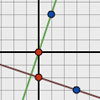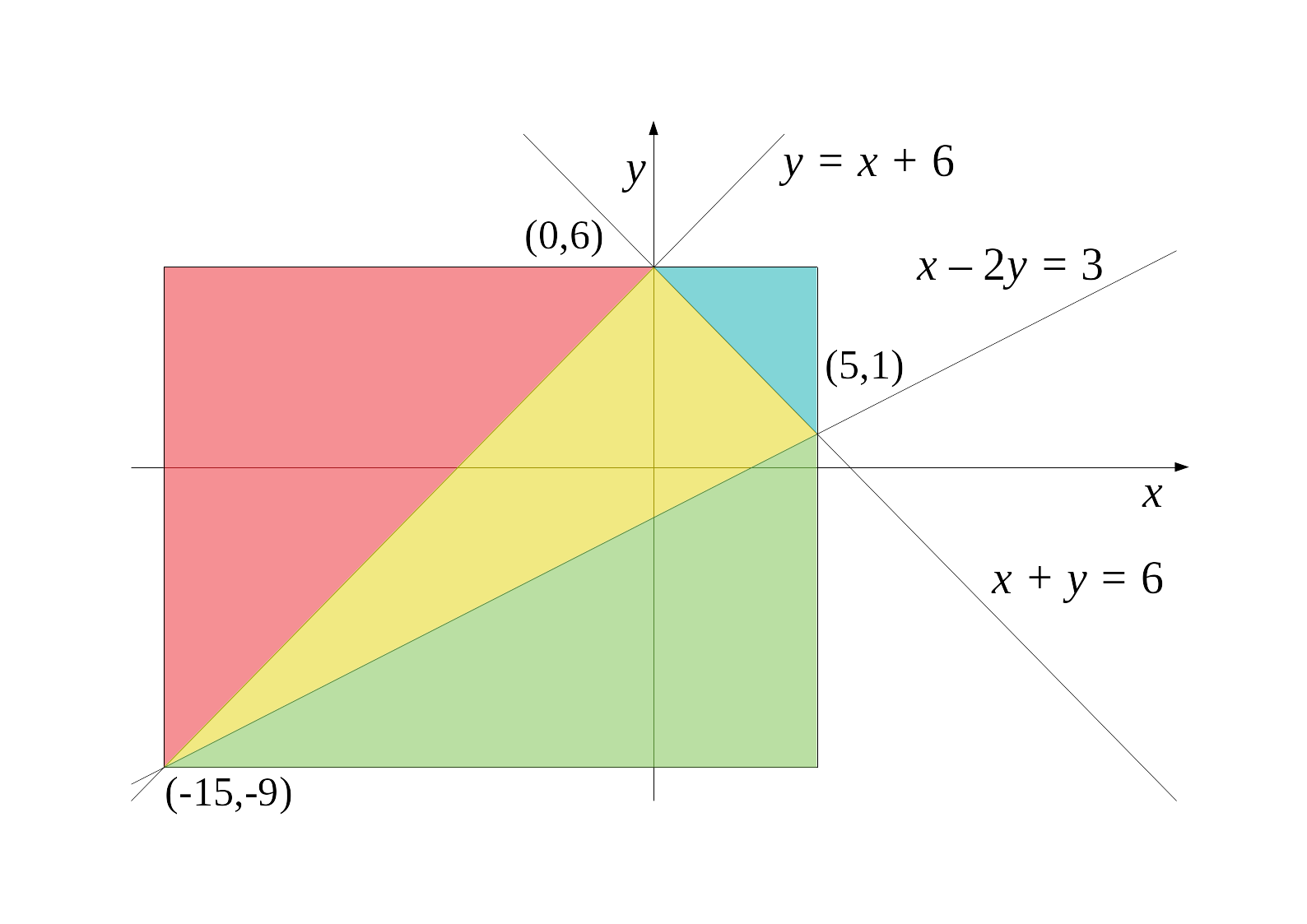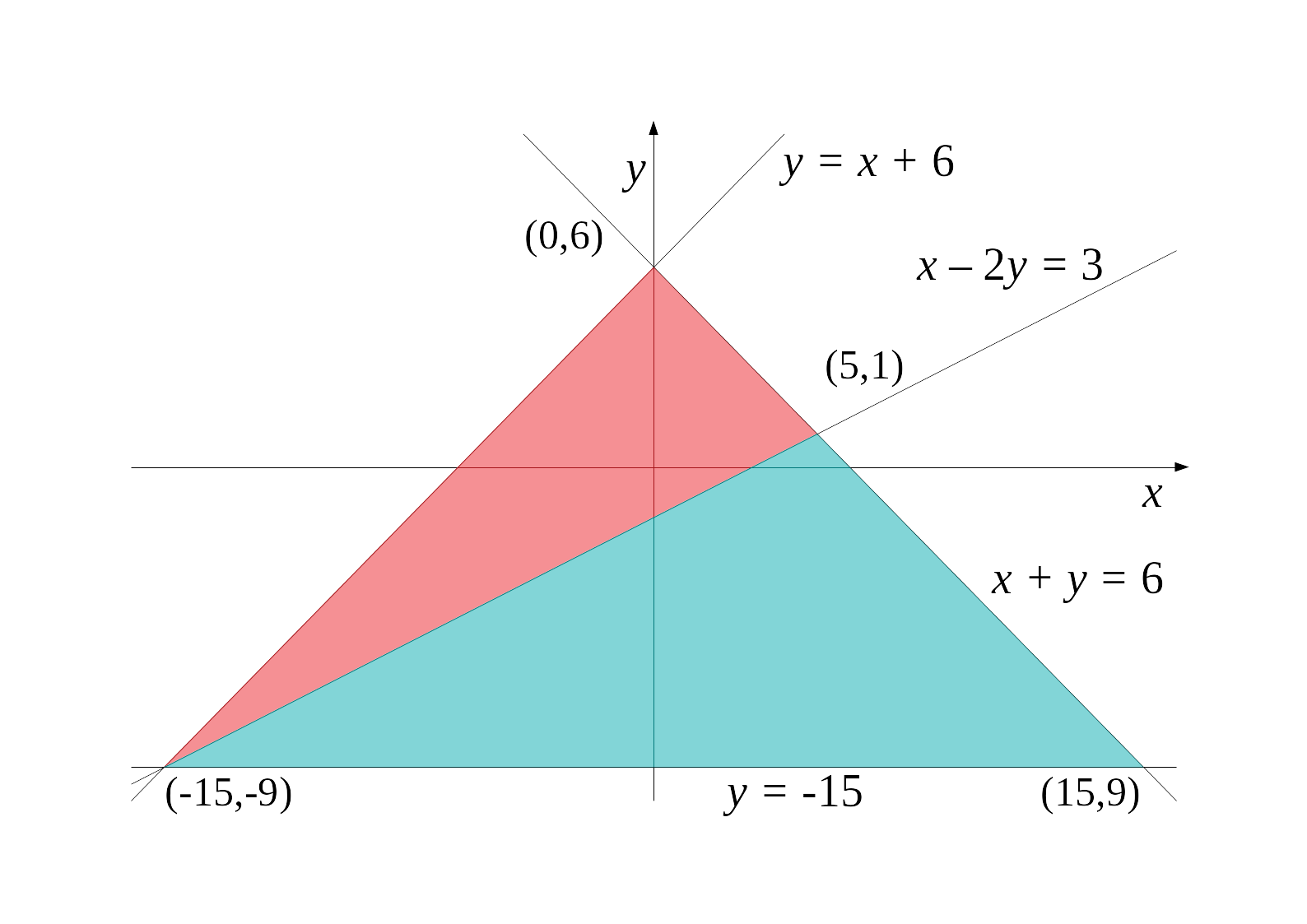#### You may also likeFour vehicles travelled on a road. What can you deduce from the times that they met?### Intersections

Change one equation in this pair of simultaneous equations very slightly and there is a big change in the solution. Why?### Perpendicular Lines

Position the lines so that they are perpendicular to each other. What can you say about the equations of perpendicular lines?

# Graphical Triangle

##### Age 14 to 16 ShortChallenge Level

The equations of the three lines must be considered in pairs to find the coordinates of their points of intersection, i.e. the coordinates of the vertices of the triangle. By solving each pair of simultaneous equations, you should find that the coordinates are $(-15,-9)$, $(0,6)$ and $(5,1)$.Subtracting Triangles From A Rectangle:
The area of the triangle may now be found by enclosing the triangle in a rectangle and subtracting the areas of the three surrounding triangles from the area of the rectangle, i.e Yellow Triangle = Rectangle - (Red Triangle + Blue Triangle + Green Triangle). This gives $300 - (112 ½ + 12 ½ + 100) = 75$.Subtracting A Triangle From A Larger Triangle:
The area of the triangle may be found by caluculating the area of the large triangle and subtracting the area of the 'extra' triangle, i.e. Red Triangle = Combined Triangle - Blue Triangle. This gives $225-150=75$.

This problem is taken from the UKMT Mathematical Challenges.
You can find more short problems, arranged by curriculum topic, in our short problems collection.